# NCERT Solutions for Class 8 Maths Chapter 4 Practical Geometry Ex 4.3

NCERT Solutions for Class 8 Maths Chapter 4 Practical Geometry Ex 4.3

### NCERT Solutions for Class 8 Maths Chapter 4 Practical Geometry Exercise 4.3

Ex 4.3 Class 8 Maths Question 1.
MO = 6 cm, ∠R = 105°, OR = 4.5 cm, ∠M = 60°, ∠O = 105°
PL = 4 cm, LA = 6.5 cm, ∠P = 90°, ∠A = 110°, ∠N = 85°
(iii) Parallelogram HEAR
HE = 5 cm, EA = 6 cm, ∠R = 85°
(iv) Rectangle OKAY
OK = 7 cm, KA = 5 cm
Solution:
(i) Construction:
Step I: Draw OR = 4.5 cm
Step II: Draw two angles of 105° each at O and R with the help of protactor.
Step III: Cut OM = 6 cm.Step IV: Draw an angle of 60° at M to meet the angle line through R at E.
Thus, MORE is the required quadrilateral.

(ii) Construction:Step I: Draw LA = 6.5 cm
Step II: Draw an angle of 75° at L and 110° at A with the help of a protractor.
[∵ 360° – (110° + 90° + 85°) = 75°]
Step III: Cut LP = 4 cm.
Step IV: Draw an angle of 90° at P which meets the angle line through A at N.
Thus PLAN is the required quadrilateral.

(iii) Construction: (Opposite sides of a parallelogram are equal)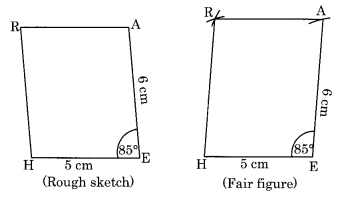Step I: Draw HE = 5 cm.
Step II: Draw an angle of 85° at E and cut EA = 6 cm.
Step III: Draw an arc with centre A and radius 5 cm.
Step IV: Draw another arc with centre H and radius 6 cm to meet the previous arc at R.
Step V: Join HR and AR
Thus, HEAR is the required parallelogram.

(iv) Construction:
(Each angle of a rectangle is 90° and opposite sides are equal.)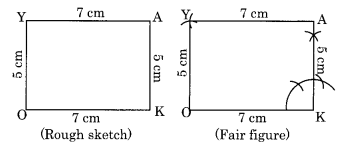Step I: Draw OK = 7 cm.
Step II: Draw the angle of 90° at K and cut KA = 5 cm.
Step III: Draw an arc with centre O and radius 5 cm.
Step IV: Draw another arc with centre A and radius 7 cm to meet the previous arc at Y.
Step V: Join OY and AY.
Thus OKAY is the required rectangle.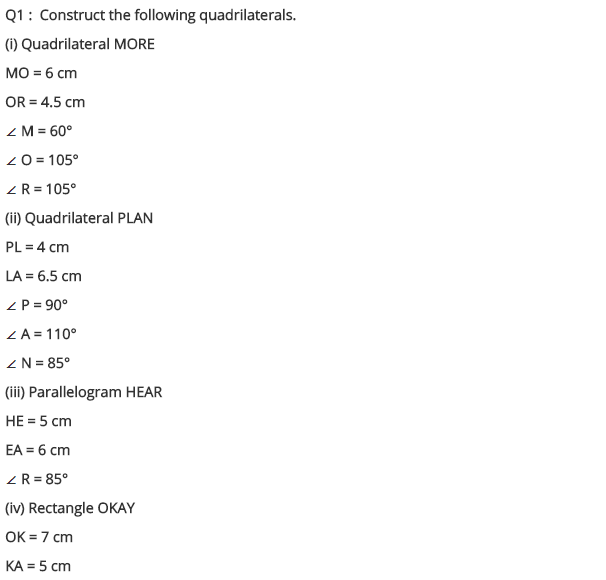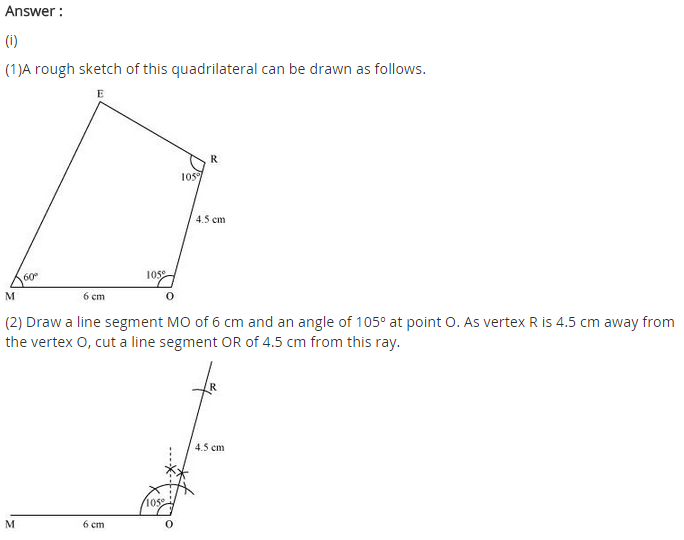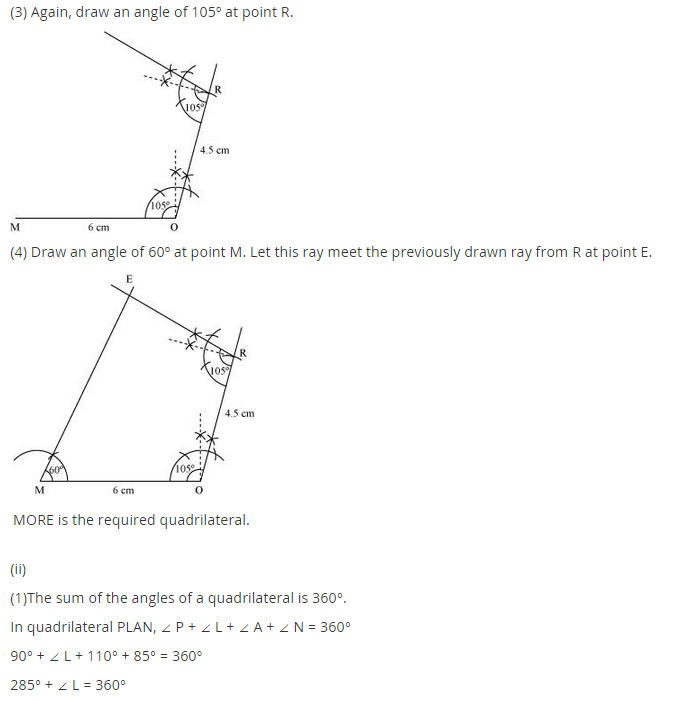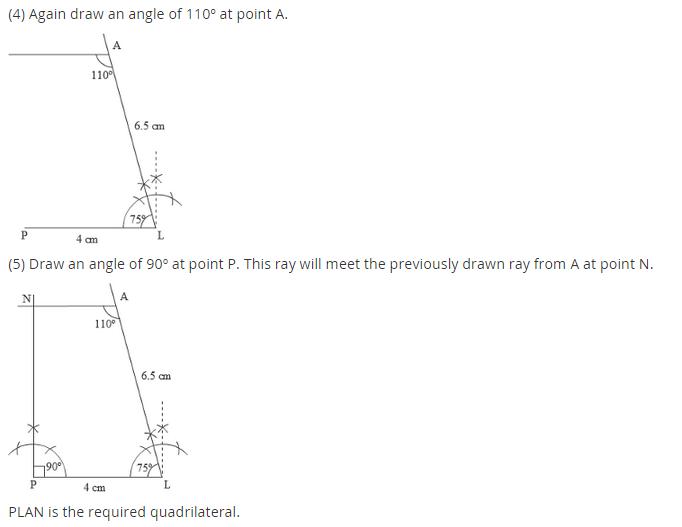## SabDekho

The Complete Educational Website